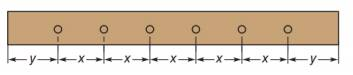Chapter A.1, Problem 25EElementary Geometry For College St...

7th Edition
Alexander + 2 others
ISBN: 9781337614085

Solutions

Chapter
SectionElementary Geometry For College St...

7th Edition
Alexander + 2 others
ISBN: 9781337614085
Textbook Problem

Using x and y, find an expression for the length of the pegged board shown in the accompanying figure.To determine

To find:

The expression for the length of the pegged board.

Explanation

1) Like terms are terms that contain exactly the same variables raised to exactly the same powers.

2) Two like terms can be added or subtracted in any polynomial.

Calculation:

The length of the pegged board is equal to y + x + x + x + x + x + y.

Add like terms in y + x + x + x + x + x + y...

Still sussing out bartleby?

Check out a sample textbook solution.

See a sample solution

The Solution to Your Study Problems

Bartleby provides explanations to thousands of textbook problems written by our experts, many with advanced degrees!

Get Started

limx0xex=1

Single Variable Calculus: Early Transcendentals, Volume I

3. If what is

Mathematical Applications for the Management, Life, and Social Sciences

Convert the expressions in Exercises 6584 to power form. 25x3

Finite Mathematics and Applied Calculus (MindTap Course List)

True or False: If F is conservative, then curl F = 0.

Study Guide for Stewart's Multivariable Calculus, 8th